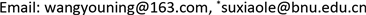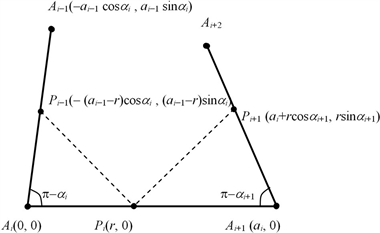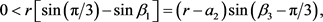﻿ 正多边形等截分之逆问题 The Inverse Question of the Equal Segmentation Problem of Regular Polygons

Pure Mathematics
Vol. 09  No. 09 ( 2019 ), Article ID: 32864 , 11 pages
10.12677/PM.2019.99126

The Inverse Question of the Equal Segmentation Problem of Regular Polygons

Youning Wang, Xiaole Su*

Laboratory of Mathematics and Complex Systems of Ministry of Education, School of Mathematical Science, Beijing Normal University, BeijingReceived: Oct. 14th, 2019; accepted: Oct. 31st, 2019; published: Nov. 7th, 2019ABSTRACT

In this paper, we focus on the inverse question of the equal segmentation problem of the regular polygons, which is a generalization of the corresponding problem for triangles and squares.

Keywords:Regular Polygon, Equal Segmentation Problem, Square, Regular Triangle1. 引言

2. 准备工作

$\underset{i=1}{\overset{n}{\sum }}{\alpha }_{i}=2\text{π}\text{.}$ (1)

$\left({a}_{i-1}-r\right)\mathrm{cos}\left(\frac{2\text{π}}{n}-{\alpha }_{i}\right)+r\left(\mathrm{cos}\frac{2\text{π}}{n}-\mathrm{cos}{\alpha }_{i+1}\right)=\left({a}_{i}-r\right),$ (2)

$\left({a}_{i-1}-r\right)\mathrm{sin}\left(\frac{2\text{π}}{n}-{\alpha }_{i}\right)+r\left(\mathrm{sin}\frac{2\text{π}}{n}-\mathrm{sin}{\alpha }_{i+1}\right)=0,$ (3)Figure 1. Sketch map of equal segmentation

${P}_{i-1}{P}_{i}\left(\begin{array}{cc}\mathrm{cos}\frac{\text{2π}}{n}& \mathrm{sin}\frac{\text{2π}}{n}\\ -\mathrm{sin}\frac{\text{2π}}{n}& \mathrm{cos}\frac{\text{2π}}{n}\end{array}\right)={P}_{i}{P}_{i+1},$ (4)

$\begin{array}{c}{a}_{i}-r+r\mathrm{cos}{\alpha }_{i+1}=\left[r+\left({a}_{i-1}-r\right)\mathrm{cos}{\alpha }_{i}\right]\mathrm{cos}\left(\text{2π}/n\right)+\left({a}_{i-1}-r\right)\mathrm{sin}{\alpha }_{i}\mathrm{sin}\left(\text{2π}/n\right)\\ =\left({a}_{i-1}-r\right)\mathrm{cos}\left(\text{2π}/n-{\alpha }_{i}\right)+r\mathrm{cos}\left(\text{2π}/n\right)\end{array}$

$\begin{array}{c}r\mathrm{sin}{\alpha }_{i+1}=\left[r+\left({a}_{i-1}-r\right)\mathrm{cos}{\alpha }_{i}\right]\mathrm{sin}\left(\text{2π}/n\right)-\left({a}_{i-1}-r\right)\mathrm{sin}{\alpha }_{i}\mathrm{cos}\left(\text{2π}/n\right)\\ =\left({a}_{i-1}-r\right)\mathrm{sin}\left(\text{2π}/n-{\alpha }_{i}\right)+r\mathrm{sin}\left(\text{2π}/n\right)\end{array}$

$\mathrm{sin}\left(\frac{2\text{π}}{n}-{\alpha }_{i}\right)=\text{0}，\text{\hspace{0.17em}}\text{\hspace{0.17em}}\text{\hspace{0.17em}}\mathrm{sin}{\alpha }_{i+1}=\mathrm{sin}\frac{2\text{π}}{n}，$

$\mathrm{cos}\left(\frac{2\text{π}}{n}-{\alpha }_{i}\right)=1，\text{\hspace{0.17em}}\text{\hspace{0.17em}}\text{\hspace{0.17em}}\mathrm{cos}{\alpha }_{i+1}=\mathrm{cos}\frac{2\text{π}}{n}$

${\alpha }_{i}=\frac{2\text{π}}{n},\text{\hspace{0.17em}}i=1,2,\cdots ,n.$

$\frac{{a}_{i-1}}{r}\mathrm{sin}\left(\frac{2\text{π}}{n}-{\alpha }_{i}\right)-\mathrm{sin}\left(\frac{2\text{π}}{n}-{\alpha }_{i}\right)+\mathrm{sin}\frac{2\text{π}}{n}-\mathrm{sin}{\alpha }_{i+1}=0,$ (5)

$\mathrm{sin}{\alpha }_{i-1}\mathrm{sin}\left(\frac{2\text{π}}{n}-{\alpha }_{i}\right)-\mathrm{sin}{\alpha }_{i}\mathrm{sin}\left(\frac{\text{4π}}{n}-{\alpha }_{i-1}-{\alpha }_{i}\right)+\mathrm{sin}{\alpha }_{i+1}\mathrm{sin}\left(\frac{2\text{π}}{n}-{\alpha }_{i-1}\right)=0,$ (6)

$\left({a}_{i-1}-r\right)\mathrm{cos}\left(\frac{2\text{π}}{n}-{\alpha }_{i}\right)-\left({a}_{i}-r\right)+r\left(\mathrm{cos}\frac{2\text{π}}{n}-\mathrm{cos}{\alpha }_{i+1}\right)=0,$ (7)

$\left({a}_{i}-r\right)\mathrm{sin}\left(\frac{2\text{π}}{n}-{\alpha }_{i+1}\right)+r\left(\mathrm{sin}\frac{2\text{π}}{n}-\mathrm{sin}{\alpha }_{i+\text{2}}\right)=0.$ (8)

$\begin{array}{c}\text{0}=|\begin{array}{ccc}\mathrm{cos}\left(\frac{2\text{π}}{n}-{\alpha }_{i}\right)& -1& \mathrm{cos}\frac{2\text{π}}{n}-\mathrm{cos}{\alpha }_{i+1}\\ \mathrm{sin}\left(\frac{2\text{π}}{n}-{\alpha }_{i}\right)& 0& \mathrm{sin}\frac{2\text{π}}{n}-\mathrm{sin}{\alpha }_{i+1}\\ 0& \mathrm{sin}\left(\frac{2\text{π}}{n}-{\alpha }_{i+1}\right)& \mathrm{sin}\frac{2\text{π}}{n}-\mathrm{sin}{\alpha }_{i+2}\end{array}|\\ =|\begin{array}{cc}\mathrm{sin}\left(\frac{2\text{π}}{n}-{\alpha }_{i}\right)& \mathrm{sin}\frac{2\text{π}}{n}-\mathrm{sin}{\alpha }_{i+1}\\ 0& \mathrm{sin}\frac{2\text{π}}{n}-\mathrm{sin}{\alpha }_{i+2}\end{array}|-\mathrm{sin}\left(\frac{2\text{π}}{n}-{\alpha }_{i+1}\right)|\begin{array}{cc}\mathrm{cos}\left(\frac{2\text{π}}{n}-{\alpha }_{i}\right)& \mathrm{cos}\frac{2\text{π}}{n}-\mathrm{cos}{\alpha }_{i+1}\\ \mathrm{sin}\left(\frac{2\text{π}}{n}-{\alpha }_{i}\right)& \mathrm{sin}\frac{2\text{π}}{n}-\mathrm{sin}{\alpha }_{i+1}\end{array}|\\ =\mathrm{sin}\frac{2\text{π}}{n}\mathrm{sin}\left(\frac{2\text{π}}{n}-{\alpha }_{i}\right)-\mathrm{sin}\left(\frac{2\text{π}}{n}-{\alpha }_{i}\right)\mathrm{sin}{\alpha }_{i+2}\\ \text{\hspace{0.17em}}\text{\hspace{0.17em}}-\mathrm{sin}\left(\frac{2\text{π}}{n}-{\alpha }_{i+1}\right)\left[\mathrm{sin}\frac{2\text{π}}{n}\mathrm{cos}\left(\frac{2\text{π}}{n}-{\alpha }_{i}\right)-\mathrm{cos}\frac{2\text{π}}{n}\mathrm{sin}\left(\frac{2\text{π}}{n}-{\alpha }_{i}\right)\right]\\ \text{\hspace{0.17em}}\text{\hspace{0.17em}}-\mathrm{sin}\left(\frac{2\text{π}}{n}-{\alpha }_{i+1}\right)\left[-\mathrm{cos}\left(\frac{2\text{π}}{n}-{\alpha }_{i}\right)\mathrm{sin}{\alpha }_{i+1}+\mathrm{sin}\left(\frac{2\text{π}}{n}-{\alpha }_{i}\right)\mathrm{cos}{\alpha }_{i+1}\right]\end{array}$

$\begin{array}{l}=-\mathrm{sin}{\alpha }_{i}\mathrm{sin}\left(\frac{2\text{π}}{n}-{\alpha }_{i+1}\right)-\mathrm{sin}{\alpha }_{i+2}\mathrm{sin}\left(\frac{2\text{π}}{n}-{\alpha }_{i}\right)+\mathrm{sin}\left(\frac{2\text{π}}{n}-{\alpha }_{i}\right)\mathrm{sin}\left(\frac{2\text{π}}{n}-{\alpha }_{i+1}+{\alpha }_{i+1}\right)\\ \text{\hspace{0.17em}}\text{\hspace{0.17em}}+\mathrm{cos}\left(\frac{2\text{π}}{n}-{\alpha }_{i}\right)\mathrm{sin}\left(\frac{2\text{π}}{n}-{\alpha }_{i+1}\right)\mathrm{sin}{\alpha }_{i+1}-\mathrm{sin}\left(\frac{2\text{π}}{n}-{\alpha }_{i}\right)\mathrm{sin}\left(\frac{2\text{π}}{n}-{\alpha }_{i+1}\right)\mathrm{cos}{\alpha }_{i+1}\\ =-\mathrm{sin}{\alpha }_{i}\mathrm{sin}\left(\frac{2\text{π}}{n}-{\alpha }_{i+1}\right)-\mathrm{sin}{\alpha }_{i+2}\mathrm{sin}\left(\frac{2\text{π}}{n}-{\alpha }_{i}\right)\\ \text{\hspace{0.17em}}\text{\hspace{0.17em}}+\mathrm{cos}\left(\frac{2\text{π}}{n}-{\alpha }_{i}\right)\mathrm{sin}\left(\frac{2\text{π}}{n}-{\alpha }_{i+1}\right)\mathrm{sin}{\alpha }_{i+1}+\mathrm{sin}\left(\frac{2\text{π}}{n}-{\alpha }_{i}\right)\mathrm{cos}\left(\frac{2\text{π}}{n}-{\alpha }_{i+1}\right)\mathrm{sin}{\alpha }_{i+1}\\ =-\mathrm{sin}{\alpha }_{i}\mathrm{sin}\left(\frac{2\text{π}}{n}-{\alpha }_{i+1}\right)-\mathrm{sin}{\alpha }_{i+2}\mathrm{sin}\left(\frac{2\text{π}}{n}-{\alpha }_{i}\right)+\mathrm{sin}{\alpha }_{i+1}\mathrm{sin}\left(\frac{4\text{π}}{n}-{\alpha }_{i}-{\alpha }_{i+1}\right)\end{array}$

$\left({a}_{j-1}-r\right)\mathrm{cos}\left(\frac{2\text{π}}{n}-{\alpha }_{j}\right)={a}_{j}-r,\text{\hspace{0.17em}}\text{\hspace{0.17em}}\text{\hspace{0.17em}}\left({a}_{j-1}-r\right)\mathrm{sin}\left(\frac{2\text{π}}{n}-{\alpha }_{j}\right)=0,$

${a}_{j}+r\left(\mathrm{cos}\frac{2\text{π}}{n}-\mathrm{cos}{\alpha }_{j+2}\right)={a}_{j+1},\text{\hspace{0.17em}}\text{\hspace{0.17em}}\text{\hspace{0.17em}}r\left(\mathrm{sin}\frac{2\text{π}}{n}-\mathrm{sin}{\alpha }_{j+2}\right)=0.$

${\alpha }_{j+2}=\frac{2\text{π}}{n},\text{\hspace{0.17em}}\text{\hspace{0.17em}}\text{\hspace{0.17em}}{a}_{j}={a}_{j+1}.$

${\alpha }_{j+2}=\frac{2\text{π}}{n},\text{\hspace{0.17em}}\text{\hspace{0.17em}}\text{\hspace{0.17em}}{a}_{j}={a}_{j+1}.$

$\mathrm{sin}\left(\frac{2\text{π}}{n}-{\alpha }_{j}\right)=0,$

${\alpha }_{j}=\frac{2\text{π}}{n},\text{\hspace{0.17em}}\text{\hspace{0.17em}}\text{\hspace{0.17em}}{a}_{j-1}={a}_{j}$

$\left({a}_{j-1}-r\right)\mathrm{cos}\left(\frac{2\text{π}}{n}-{\alpha }_{j}\right)=\left({a}_{j}-r\right),$

$\left({a}_{j-1}-r\right)\mathrm{sin}\left(\frac{2\text{π}}{n}-{\alpha }_{j}\right)=0.$

$00|i=1,2,\cdots ,n\right\}$$r>\mathrm{max}\left\{{a}_{i}>0|i=1,2,\cdots ,n\right\}>0$，则

$\mathrm{cos}\left(\frac{2\text{π}}{n}-{\alpha }_{j}\right)>0,\text{\hspace{0.17em}}\text{\hspace{0.17em}}\text{\hspace{0.17em}}\mathrm{sin}\left(\frac{2\text{π}}{n}-{\alpha }_{j}\right)=0.$

3. 主要结果

$\text{0}=\mathrm{sin}{\alpha }_{j-1}\mathrm{sin}\left(\frac{2\text{π}}{n}-{\alpha }_{j}\right)+\mathrm{sin}{\alpha }_{j+1}\mathrm{sin}\left(\frac{2\text{π}}{n}-{\alpha }_{j-1}\right)=\left(\mathrm{sin}{\alpha }_{j-1}-\mathrm{sin}{\alpha }_{j+1}\right)\mathrm{sin}\left(\frac{2\text{π}}{n}-{\alpha }_{j}\right),$

$\frac{{a}_{i-1}}{r}=\text{1}-\frac{\mathrm{sin}\frac{2\text{π}}{n}-\mathrm{sin}{\alpha }_{i+1}}{\mathrm{sin}\left(\frac{2\text{π}}{n}-{\alpha }_{i}\right)}=\text{1}-\frac{\mathrm{sin}\frac{2\text{π}}{n}-\mathrm{sin}\left(\frac{4\text{π}}{n}-{\alpha }_{i}\right)}{\mathrm{sin}\left(\frac{2\text{π}}{n}-{\alpha }_{i}\right)}=2{\mathrm{cos}}^{\text{2}}\frac{\text{π}}{n}\left[\text{1}-\mathrm{tan}\frac{\text{π}}{n}\mathrm{tan}\left(\frac{\text{π}}{n}-\frac{{\alpha }_{i}}{2}\right)\right],$

$2\mathrm{cos}\frac{\text{2π}}{n}<\frac{{a}_{i}}{r}<\text{2,}$

$\frac{{a}_{i-1}+{a}_{i}}{r}=2{\mathrm{cos}}^{2}\frac{\text{π}}{n}\left[2-\mathrm{tan}\frac{\text{π}}{n}\mathrm{tan}\left(\frac{\text{π}}{n}-\frac{{\alpha }_{i}}{2}\right)-\mathrm{tan}\frac{\text{π}}{n}\mathrm{tan}\left(\frac{\text{π}}{n}-\frac{{\alpha }_{i+1}}{2}\right)\right]=4{\mathrm{cos}}^{2}\frac{\text{π}}{n},$

$\frac{{a}_{i}}{r}>2\mathrm{cos}\frac{\text{2π}}{n}\left\{\begin{array}{l}=0,n=4;\\ \ge 1,n\ge 6.\end{array}$

$1-\frac{{a}_{i-1}}{r}=\frac{\mathrm{sin}\frac{2\text{π}}{n}-\mathrm{sin}{\alpha }_{i+1}}{\mathrm{sin}\left(\frac{2\text{π}}{n}-{\alpha }_{i}\right)},$ (9)

$\frac{\mathrm{sin}\frac{2\text{π}}{n}-\mathrm{sin}{\alpha }_{i+1}}{\mathrm{sin}\left(\frac{2\text{π}}{n}-{\alpha }_{i}\right)}<0，$ (10)

$\mathrm{sin}\frac{2\text{π}}{n}-\mathrm{sin}{\alpha }_{i+1}=\left(1-\frac{{a}_{i-1}}{r}\right)\mathrm{sin}\left(\frac{2\text{π}}{n}-{\alpha }_{i}\right),$ (11)

$\mathrm{sin}\frac{\text{π}}{\text{3}}-\mathrm{sin}{\beta }_{i+1}=\left(1-\frac{{a}_{i-1}}{r}\right)\mathrm{sin}\left({\beta }_{i}-\frac{\text{π}}{\text{3}}\right).$ (12)

$\mathrm{sin}\frac{2\text{π}}{n}-\mathrm{sin}{\alpha }_{\text{2}}=\left(1-\frac{{a}_{\text{0}}}{r}\right)\mathrm{sin}\left(\frac{2\text{π}}{n}-{\alpha }_{\text{1}}\right)\ge \text{0,}$

${\alpha }_{2}\in \left(0,\text{2π}/n\right]\cup \left[\left(n-\text{2}\right)\text{π}/n,\text{π}\right).$

$\mathrm{sin}\frac{2\text{π}}{n}-\mathrm{sin}{\alpha }_{\text{3}}=\left(1-\frac{{a}_{\text{1}}}{r}\right)\mathrm{sin}\left(\frac{2\text{π}}{n}-{\alpha }_{\text{2}}\right)\le \text{0,}$

${\alpha }_{3}\in \left[\text{2π}/n,\left(n-\text{2}\right)\text{π}/n\right],$

$\cdots$

$\mathrm{sin}\frac{2\text{π}}{n}-\mathrm{sin}{\alpha }_{i+1}=\left(1-\frac{{a}_{i-1}}{r}\right)\mathrm{sin}\left(\frac{2\text{π}}{n}-{\alpha }_{i}\right)\le \text{0,}$

${\alpha }_{i+1}\in \left[\text{2π}/n,\left(n-\text{2}\right)\text{π}/n\right],$

$\cdots$

$\mathrm{sin}\frac{2\text{π}}{n}-\mathrm{sin}{\alpha }_{\text{1}}=\left(1-\frac{{a}_{n-1}}{r}\right)\mathrm{sin}\left(\frac{2\text{π}}{n}-{\alpha }_{n}\right)\le \text{0,}$

${\alpha }_{1}\in \left[\text{2π}/n,\left(n-\text{2}\right)\text{π}/n\right],$

${\alpha }_{1}\in \left[\text{2π}/n,\left(n-\text{2}\right)\text{π}/n\right]\cap \left(0,\text{2π}/n\right]=\left\{\text{2π}/n\right\},$

${\alpha }_{i}=\text{2π}/n,i=1,2,\cdots ,n$

${a}_{i}={a}_{i-1},i=1,2,\cdots ,n$

$\mathrm{sin}\frac{\text{π}}{\text{3}}-\mathrm{sin}{\beta }_{2}=\left(1-\frac{{a}_{0}}{r}\right)\mathrm{sin}\left({\beta }_{1}-\frac{\text{π}}{\text{3}}\right)\le 0,$

${\beta }_{2}\in \left[\text{π}/3,\text{2π}/3\right],$

$\mathrm{sin}\frac{\text{π}}{\text{3}}-\mathrm{sin}{\beta }_{3}=\left(1-\frac{{a}_{1}}{r}\right)\mathrm{sin}\left({\beta }_{2}-\frac{\text{π}}{\text{3}}\right)\ge 0,$

${\beta }_{3}\in \left(0,\text{π}/3\right]\cup \left[\text{2π}/3,\text{π}\right).$

${\beta }_{3}\in \left(0,\text{π}/3\right].$

$\mathrm{sin}\frac{\text{π}}{\text{3}}-\mathrm{sin}{\beta }_{\text{1}}=\left(1-\frac{{a}_{\text{2}}}{r}\right)\mathrm{sin}\left({\beta }_{\text{3}}-\frac{\text{π}}{\text{3}}\right)\le 0,$

${\beta }_{1}\in \left[\text{π}/3,\text{2π}/3\right],$

${\beta }_{1}\in \left[\text{π}/3,\text{2π}/3\right]\cap \left(0,\text{π}/3\right]=\left\{\text{π}/3\right\}.$

$0\le r\left[\mathrm{sin}\left(\text{π}/3\right)-\mathrm{sin}{\beta }_{1}\right]=\left(r-{a}_{2}\right)\mathrm{sin}\left({\beta }_{3}-\text{π}/3\right)\le 0,$

${\beta }_{1}=\text{π}/3,$

$f\left({\beta }_{1},{\beta }_{2}\right)=\mathrm{sin}{\beta }_{1}\mathrm{sin}\left(\text{π}/3-{\beta }_{2}\right)-\mathrm{sin}{\beta }_{2}\mathrm{sin}\left(\text{π}/3+{\beta }_{1}+{\beta }_{2}\right)+\mathrm{sin}\left({\beta }_{1}+{\beta }_{2}\right)\mathrm{sin}\left(\text{π}/3-{\beta }_{1}\right),$

$f\left(0,{\beta }_{2}\right)=\mathrm{sin}{\beta }_{2}\left[\mathrm{sin}\left(\text{π}/3\right)-\mathrm{sin}\left(\text{π}/3+{\beta }_{2}\right)\right],$

$f\left({\beta }_{1},\text{π}/3-{\beta }_{1}\right)={\mathrm{sin}}^{2}{\beta }_{1},$

$\begin{array}{c}f\left({\beta }_{1},{\beta }_{1}\right)=\mathrm{sin}{\beta }_{1}\mathrm{sin}\left(\text{π}/3-{\beta }_{1}\right)-\mathrm{sin}{\beta }_{1}\mathrm{sin}\left(\text{π}/3+2{\beta }_{1}\right)+\mathrm{sin}\left(2{\beta }_{1}\right)\mathrm{sin}\left(\text{π}/3-{\beta }_{1}\right)\\ =\mathrm{sin}{\beta }_{1}\left[\mathrm{sin}\left(\text{π}/3-{\beta }_{1}\right)-\mathrm{sin}\left(\text{3π}/3-2{\beta }_{1}\right)+2\mathrm{cos}{b}_{1}\mathrm{sin}\left(\text{π}/3-{\beta }_{1}\right)\right]\\ =2\mathrm{sin}{\beta }_{1}\mathrm{sin}\left(\text{π}/3-{\beta }_{1}\right)\left[\mathrm{cos}\left(\text{π}/3\right)-\mathrm{cos}\left(\text{π}/3-{\beta }_{1}\right)+\mathrm{cos}{\beta }_{1}\right]\\ =2\mathrm{sin}{\beta }_{1}\mathrm{sin}\left(\text{π}/3-{\beta }_{1}\right)\left[\mathrm{cos}\left(\text{π}/3\right)+\mathrm{cos}\left(\text{π}/3+{\beta }_{1}\right)\right]\end{array}$

$f\left(0,{\beta }_{2}\right)<0,\text{\hspace{0.17em}}\text{\hspace{0.17em}}\text{\hspace{0.17em}}\forall {\beta }_{2}\in \left(0,\text{π}/3\right),$

$f\left({\beta }_{1},\text{π}/3-{\beta }_{1}\right)>0,\text{\hspace{0.17em}}\text{\hspace{0.17em}}\text{\hspace{0.17em}}\forall {\beta }_{1}\in \left(0,\text{π}/3\right),$

$f\left({\beta }_{1},{\beta }_{1}\right)>0,\text{\hspace{0.17em}}\text{\hspace{0.17em}}\text{\hspace{0.17em}}\forall {\beta }_{1}\in \left(0,\text{π}/3\right),$

$\begin{array}{l}f\left({\beta }_{1},\text{π}/2-{\beta }_{1}/2\right)\\ =\mathrm{sin}{\beta }_{1}\mathrm{sin}\left({\beta }_{1}/2-\text{π}/6\right)-\mathrm{cos}\left({\beta }_{1}/2\right)\mathrm{sin}\left(\text{π}/6-{\beta }_{1}/2\right)+\mathrm{cos}\left({\beta }_{1}/2\right)\mathrm{sin}\left(\text{π}/3-{\beta }_{1}\right)\\ =\mathrm{sin}\left(\text{π}/6-{\beta }_{1}/2\right)\mathrm{cos}\left({\beta }_{1}/2\right)\left[-2\mathrm{sin}\left({\beta }_{1}/2\right)-1+2\mathrm{cos}\left(\text{π}/6-{\beta }_{1}/2\right)\right]\\ =\mathrm{sin}\left(\text{π}/6-{\beta }_{1}/2\right)\mathrm{cos}\left({\beta }_{1}/2\right)\left[2\mathrm{cos}\left(\text{π}/6+{\beta }_{1}/2\right)-1\right]>0,\text{\hspace{0.17em}}\text{\hspace{0.17em}}\text{\hspace{0.17em}}\forall {\beta }_{1}\in \left(0,\text{π}/3\right)\end{array}$$r>{a}_{2}.$

$0>r\left[\mathrm{sin}\left(\text{π}/3\right)-\mathrm{sin}{\beta }_{2}\right]=\left(r-{a}_{3}\right)\mathrm{sin}\left({\beta }_{1}-\text{π}/3\right),$

$r>{a}_{3}.$

$r<{a}_{1}.$

${\beta }_{2}<\angle {P}_{2}{P}_{1}{A}_{1}<\angle {P}_{2}{P}_{1}{P}_{3}=\text{π}/3,$

${\beta }_{2}\in \left({\beta }_{1},\text{π}/2\right)-\left\{\text{π}/3\right\}-\left(\text{π}/3,\text{π}/2\right)=\left({\beta }_{1},\text{π}/3\right),$

$0

$r<{a}_{3}.$

$\begin{array}{c}{a}_{1}/{a}_{3}=\mathrm{sin}{\beta }_{3}/\mathrm{sin}{\beta }_{2}=\mathrm{sin}\left({\beta }_{1}+{\beta }_{2}\right)/\mathrm{sin}{\beta }_{2}=\mathrm{sin}{\beta }_{1}\mathrm{cos}{\beta }_{2}/\mathrm{sin}{\beta }_{2}+\mathrm{cos}{\beta }_{1}\\ >\mathrm{sin}{\beta }_{1}\mathrm{cos}\left(\text{π}/3\right)/\mathrm{sin}\left(\text{π}/3\right)+\mathrm{cos}{\beta }_{1}=\mathrm{sin}\left({\beta }_{1}+\text{π}/3\right)/\mathrm{sin}\left(\text{π}/3\right)>1\end{array}$

$r<{a}_{3}<{a}_{1},$

$r\left[\mathrm{sin}\left(\text{π}/3\right)-\mathrm{sin}{\beta }_{3}\right]=\left(r-{a}_{1}\right)\mathrm{sin}\left({\beta }_{2}-\text{π}/3\right)>0,$

${\beta }_{3}\in \left(0,\text{π}/3\right)\cup \left(\text{2π}/3,\text{π}\right).$

${\beta }_{3}\in \left[\left(0,\text{π}/3\right)\cup \left(\text{2π}/3,\text{π}\right)\right]\cap \left(\text{π}/3,\text{π}\right)=\left(\text{2π}/3,\text{π}\right).$

The Inverse Question of the Equal Segmentation Problem of Regular Polygons[J]. 理论数学, 2019, 09(09): 998-1008. https://doi.org/10.12677/PM.2019.99126

1. 1. 张慧欣. 一个几何问题的思考[J]. 数学通报, 2012, 51(11): 53-54.

2. 2. 张新. 对正三角形等截分逆问题的再思考[J]. 数学通报, 2015, 54(5): 59-60.

3. 3. 王幼宁. 正三角形等截分逆问题的推广及其解析求解[J]. 数学通报, 2016, 55(5): 51-53.

4. 4. 王幼宁, 苏效乐. 正方形等截分之逆问题[J]. 理论数学, 2019, 9(3): 386-392.